# CLIJ2GPU accelerated image processing for everyone

CLIJ2 home

# Spot detection

For counting cells, their segmentation is often not necessary. Instead, the spot-detection approach is much simpler and is easier to implement. This online lecture explains the matter more deeply.

We start by cleaning up and opening an example image. For this, we use ImageJ-functions:

```// clean up first
run("Close All");
run("Clear Results");

// open the blobs exmple image
open("http://imagej.nih.gov/ij/images/blobs.gif");
run("Invert LUT");
run("32-bit"); // we use 32 bit because negative values may appear in the workflow below.
```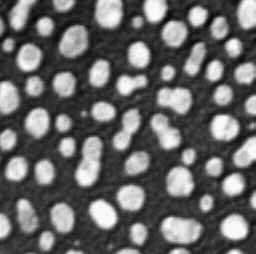We now initialize the GPU and push the image as explained in the basics of clij tutorial.

```run("CLIJ2 Macro Extensions", "cl_device=");
Ext.CLIJ2_clear();

// push image to GPU memory
blobs = getTitle();
Ext.CLIJ2_push(blobs);
```

# Detecting maxima

A simple local-maxima detection result can be seen as a binary image where all pixels are white (value=1) that had in the original image no other pixel with higher value in their neighborhood specified by a given radius. All other pixels will be black (value=0).

```radius = 1;
Ext.CLIJ2_pull(maxima);
```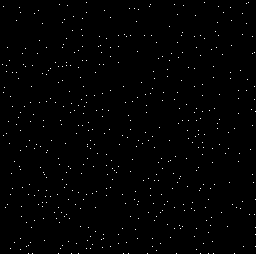For improved visualisation, we merge the blobs image with the maxima and use the HiLo lookup table to highlight where maxima were found.

```Ext.CLIJx_visualizeOutlinesOnOriginal(blobs, maxima, visualization);
Ext.CLIJ2_pull(visualization);
// configure visualization
Ext.CLIJ2_getMaximumOfAllPixels(visualization, max_intensity);
setMinAndMax(-1, max_intensity);
run("HiLo");
```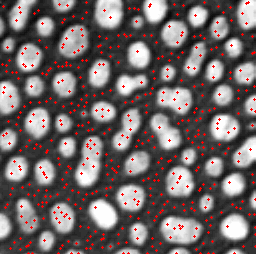Obviously, too many maxima are detectd. Furthermore, just a note: The visualization image should not be used for further analysis as pixel values were overwritten.

## Difference of Gaussian

We can use the Difference of Gaussian (DoG) filter to produce an image where only maxima of a given prominence are left for spot detection. The DoG filter applies two Gaussian blur filters to an image and subtracts the results from each other. Typically, the sigma for the first Gaussian blur should be smaller than the radius of the objects we are interested in. This filter practically does noise-removal. The second sigma, defining the Gaussian blurred image which is subtracted from the first, should be higher than the objects radius. Thus, the subtraction of this image can be seen as background subtraction. Thus, DoG is a technique combining noise and background removal.

We will apply DoG now with some random sigma values and observe visually less local maxima being present.

```sigma1 = 15;
sigma2 = 20;
Ext.CLIJ2_differenceOfGaussian2D(blobs, DoG_image, sigma1, sigma1, sigma2, sigma2);
Ext.CLIJ2_pull(DoG_image);
```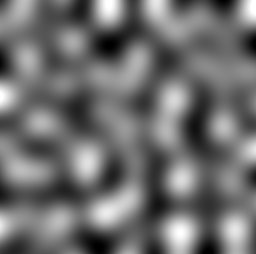We then do maxima detection and result visualization as above.

```// detect maxima

// combine with input image
Ext.CLIJx_visualizeOutlinesOnOriginal(DoG_image, maxima, visualization);

// visulize result
Ext.CLIJ2_pull(visualization);
// configure visualization
Ext.CLIJ2_getMaximumOfAllPixels(visualization, max_intensity);
setMinAndMax(-1, max_intensity);
run("HiLo");
```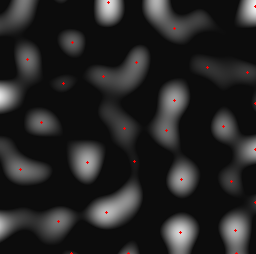Obviously, our sigma values were too high and we lost some maxima of interest while applying the DoG method.

We will try the same procedure again in a for loop to see which sigma values work well for our purpose. This time, we visualize the found maxima on the original blobs image.

```for (sigma = 3; sigma < 20; sigma += 3) {

// apply Difference of Gaussian
sigma1 = sigma - 2;
sigma2 = sigma + 2;
Ext.CLIJ2_differenceOfGaussian2D(blobs, DoG_image, sigma1, sigma1, sigma2, sigma2);

// detect maxima

// combine with input image
Ext.CLIJx_visualizeOutlinesOnOriginal(blobs, maxima, visualization);

// visulize result
Ext.CLIJ2_pull(visualization);
// configure visualization
Ext.CLIJ2_getMaximumOfAllPixels(visualization, max_intensity);
setMinAndMax(-1, max_intensity);
run("HiLo");

print("Used sigmas: ", sigma1, sigma2);
```
```> Used sigmas:  1 5
```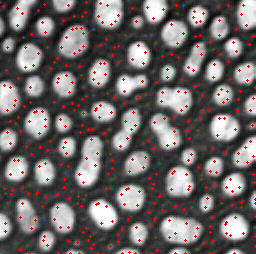```}
```
```> Used sigmas:  4 8
```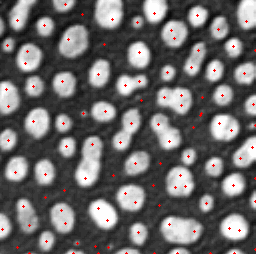```}
```
```> Used sigmas:  7 11
```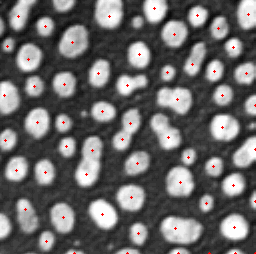```}
```
```> Used sigmas:  10 14
```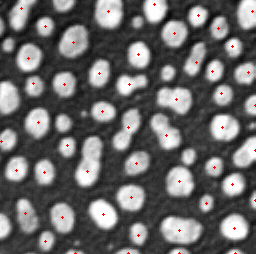```}
```
```> Used sigmas:  13 17
```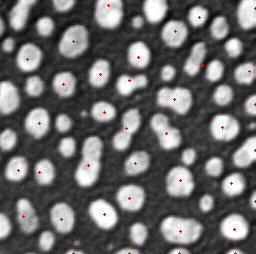```}
```
```> Used sigmas:  16 20
```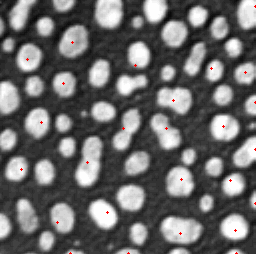```}
```

From this test, one could conclude that sigma1=7 and sigma2=11 delivers good results. This is because the objects we are interested in have a radius in that range. For exploring the parameter space a bit more in detail and also play with varius differences between sigma1 and sigma2, it is recommended to build an interactive workflow with the above shown operations using the clij assistant.

```// cleanup by the end
Ext.CLIJ2_clear();
```